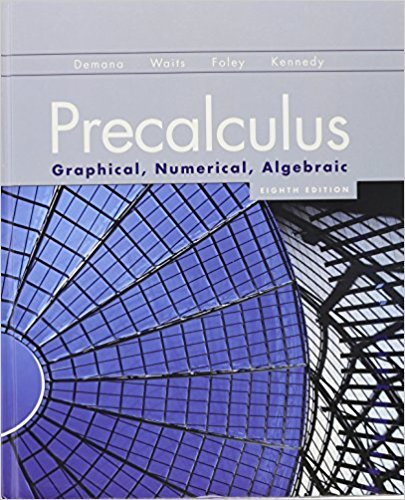×

×

Textbooks / Calculus / Precalculus: Graphical, Numerical, Algebraic 8th Edition

# Precalculus: Graphical, Numerical, Algebraic 8th Edition - Solutions by Chapter## Full solutions for Precalculus: Graphical, Numerical, Algebraic | 8th Edition

ISBN: 9780321656933Precalculus: Graphical, Numerical, Algebraic | 8th Edition - Solutions by Chapter

Solutions by Chapter
4 5 0 374 Reviews
##### ISBN: 9780321656933

This expansive textbook survival guide covers the following chapters: 88. This textbook survival guide was created for the textbook: Precalculus: Graphical, Numerical, Algebraic, edition: 8th Edition. Precalculus: Graphical, Numerical, Algebraic was written by and is associated to the ISBN: 9780321656933. The full step-by-step solution to problem in Precalculus: Graphical, Numerical, Algebraic were answered by , our top Calculus solution expert on 12/28/17, 04:31PM. Since problems from 88 chapters in Precalculus: Graphical, Numerical, Algebraic have been answered, more than 153525 students have viewed full step-by-step answer.

Key Calculus Terms and definitions covered in this textbook
• Arccotangent function

See Inverse cotangent function.

• Cardioid

A limaçon whose polar equation is r = a ± a sin ?, or r = a ± a cos ?, where a > 0.

• Convergence of a sequence

A sequence {an} converges to a if limn: q an = a

• Distance (in Cartesian space)

The distance d(P, Q) between and P(x, y, z) and Q(x, y, z) or d(P, Q) ((x ) - x 2)2 + (y1 - y2)2 + (z 1 - z 2)2

• Equilibrium point

A point where the supply curve and demand curve intersect. The corresponding price is the equilibrium price.

• Frequency (in statistics)

The number of individuals or observations with a certain characteristic.

• Grapher or graphing utility

Graphing calculator or a computer with graphing software.

• Intercept

Point where a curve crosses the x-, y-, or z-axis in a graph.

• Logarithmic form

An equation written with logarithms instead of exponents

• Modified boxplot

A boxplot with the outliers removed.

• Multiplication principle of counting

A principle used to find the number of ways an event can occur.

• Negative angle

Angle generated by clockwise rotation.

• Quotient of complex numbers

a + bi c + di = ac + bd c2 + d2 + bc - ad c2 + d2 i

• Remainder theorem

If a polynomial f(x) is divided by x - c , the remainder is ƒ(c)

• Second

Angle measure equal to 1/60 of a minute.

• Symmetric difference quotient of ƒ at a

ƒ(x + h) - ƒ(x - h) 2h

• Terminal side of an angle

See Angle.

• Trichotomy property

For real numbers a and b, exactly one of the following is true: a < b, a = b , or a > b.

• y-intercept

A point that lies on both the graph and the y-axis.

• yz-plane

The points (0, y, z) in Cartesian space.